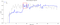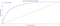# The Problem

`# Loading packagesimport pandas as pdimport numpy as npimport matplotlib.pyplot as pltimport seaborn as snsimport time%matplotlib inline# Loading the Train and Test datasetsdf_train = pd.read_csv("data/train.csv")df_test = pd.read_csv("data/test.csv")`

# 2 Basic Exploratory Analysis

`# Checking the first 5 rows of df_traindf_train.head()`
`# Checking the first 5 rows of df_testdf_test.head()`
`# Checking the genearl infos of df_train and df_testdf_train.info()`
`# Checking the genearl infos of df_testdf_test.info()`
`# Checking if is there any missing value in both train and test datasetsdf_train.isnull().sum().sum(), df_test.isnull().sum().sum()`
`# Investigating the proportion of unsatisfied customers on df_trainrate_insatisfied = df_train.TARGET.value_counts() /                                            df_train.TARGET.value_counts()rate_insatisfied * 100`

# 3 Dataset Split (train — test)

`from sklearn.model_selection import train_test_split# Spliting the dataset on a proportion of 80% for train and 20% for test.X_train, X_test, y_train, y_test = train_test_split(df_train.drop('TARGET', axis = 1), df_train.TARGET, train_size = 0.8, stratify = df_train.TARGET, random_state = 42)# Checking the splitX_train.shape, y_train.shape, X_test.shape, y_test.shape`

# 4 Feature Selection

## 4.1 Removing low variance features

`# Investigating if there are constant or semi-constat feature in X_trainfrom sklearn.feature_selection import VarianceThreshold# Removing all features that have variance under 0.01selector = VarianceThreshold(threshold = 0.01)selector.fit(X_train)mask_clean = selector.get_support()X_train = X_train[X_train.columns[mask_clean]]`
`# Total of remaning featuresX_train.shape`

## 4.2 Removing repeated features

`# Checking if there is any duplicated columnremove = []cols = X_train.columnsfor i in range(len(cols)-1):    column = X_train[cols[i]].values    for j in range(i+1,len(cols)):        if np.array_equal(column, X_train[cols[j]].values):            remove.append(cols[j])# If yes, than they will be dropped hereX_train.drop(remove, axis = 1, inplace=True)`
`# Checking if any column was droppedX_train.shape`

## 4.3 Using SelectKBest to select features

`from sklearn.feature_selection import SelectKBest, f_classif, mutual_info_classiffrom sklearn.metrics import roc_auc_score as aucfrom sklearn.model_selection import cross_val_scoreimport xgboost as xgb#Create an automated routine to test different K values in each of these methodsK_vs_score_fc = [] #List to store AUC of each K with f_classifK_vs_score_mic = [] #List to store AUC of each K with mutual_info_classifstart = time.time()for k in range(2, 247, 2):    start = time.time()        # Instantiating a KBest object for each of the metrics in order  to obtain the K features with the highest value    selector_fc = SelectKBest(score_func = f_classif, k = k)    selector_mic = SelectKBest(score_func = mutual_info_classif,     k = k)        # Selecting K-features and modifying the dataset    X_train_selected_fc = selector_fc.fit_transform(X_train,                                                                 y_train)    X_train_selected_mic = selector_mic.fit_transform(X_train, y_train)         # Instantiating an XGBClassifier object    clf = xgb.XGBClassifier(seed=42)        # Using 10-CV to calculate AUC for each K value avoinding  overfitting    auc_fc = cross_val_score(clf, X_train_selected_fc, y_train,     cv = 10, scoring = 'roc_auc')    auc_mic = cross_val_score(clf, X_train_selected_mic, y_train,    cv = 10, scoring = 'roc_auc')        # Adding the average values obtained in the CV for further  analysis.    K_vs_score_fc.append(auc_fc.mean())    K_vs_score_mic.append(auc_mic.mean())        end = time.time()    # Returning the metrics related to the tested K and the time   spent on this iteration of the loop    print("k = {} - auc_fc = {} - auc_mic = {} - Time =   {}s".format(k, auc_fc.mean(), auc_mic.mean(), end-start))        print(time.time() - start) # Computing the total time spent`
`# Ploting K_vs_score_fc e K_vs_score_mic (# of K-Best features vs AUC)import matplotlib.patches as patches# Figure setupfig, ax = plt.subplots(1, figsize = (20, 8))plt.title('Score valeus for each K', fontsize=18)plt.ylabel('Score', fontsize = 16)plt.xlabel('Value of K', fontsize = 16)ax.spines['top'].set_visible(False)ax.spines['right'].set_visible(False)plt.xticks(fontsize = 12)plt.yticks(fontsize = 12)# Create the linesplt.plot(np.arange(2, 247, 2), K_vs_score_fc, color='blue', linewidth=2)plt.plot(np.arange(2, 247, 2), K_vs_score_mic, color='grey', linewidth=2, alpha = 0.5)ax.legend(labels = ['fc', 'mic'], fontsize=14, frameon=False, loc = 'upper left')ax.set_ylim(0.80, 0.825);# Create a Rectangle patchrect = patches.Rectangle((82, 0.817), 20, (0.823 - 0.817), linewidth=2, edgecolor='r', facecolor='none')# Add the patch to the Axesax.add_patch(rect)plt.show()`Score values for both method (fc) and (mic) for the range 0,8 to 0,825.
`# Ploting the score for the best 30 featuresfeature_score = pd.Series(selector_fc.scores_, index = X_train.columns).sort_values(ascending = False)fig, ax = plt.subplots(figsize=(20, 12))ax.barh(feature_score.index[0:30], feature_score[0:30])plt.gca().invert_yaxis()ax.set_xlabel('K-Score', fontsize=18);ax.set_ylabel('Features', fontsize=18);ax.set_title('30 best features by its K-Score', fontsize = 20)plt.yticks(fontsize = 14)plt.xticks(fontsize = 14)ax.spines['top'].set_visible(False)ax.spines['right'].set_visible(False)ax.spines['left'].set_visible(False);`
`# Creating datasets where only the selected 96 features are includedX_train_selected = X_train[selected_col]X_test_selected = X_test[selected_col]`

# 5 Bayesian Optimization to the XGBClassifier

`# Function for hyperparamters tunning# Implementation learned on a lesson of Mario Filho (Kagle Grandmaster) for parametes optmization.# Link to the video: https://www.youtube.com/watch?v=WhnkeasZNHIfrom skopt import forest_minimizedef tune_xgbc(params):    """Function to be passed as scikit-optimize minimizer/maximizer   input        Parameters:    Tuples with information about the range that the optimizer should use for that parameter,     as well as the behavior that it should follow in that range.        Returns:    float: the metric that should be minimized. If the objective is maximization, then the negative     of the desired metric must be returned. In this case, the negative AUC average generated by CV is returned.    """            #Hyperparameters to be optimized    print(params)    learning_rate = params     n_estimators = params     max_depth = params    min_child_weight = params    gamma = params    subsample = params    colsample_bytree = params                #Model to be optimized     mdl = xgb.XGBClassifier(learning_rate = learning_rate,                        .   n_estimators = n_estimators, max_depth = max_depth,     min_child_weight = min_child_weight, gamma = gamma,     subsample = subsample, colsample_bytree = colsample_bytree,     seed = 42)#Cross-Validation in order to avoid overfitting    auc = cross_val_score(mdl, X_train_selected, y_train,     cv = 10,    scoring = 'roc_auc')        print(auc.mean())    # as the function is minimization (forest_minimize), we need to use the negative of the desired metric (AUC)    return -auc.mean()`
`# Creating a sample space in which the initial randomic search should be performedspace = [(1e-3, 1e-1, 'log-uniform'), # learning rate          (100, 2000), # n_estimators          (1, 10), # max_depth           (1, 6.), # min_child_weight           (0, 0.5), # gamma           (0.5, 1.), # subsample           (0.5, 1.)] # colsample_bytree# Minimization using a random forest with 20 random samples and 50 iterations for Bayesian optimization.result = forest_minimize(tune_xgbc, space, random_state=42, n_random_starts=20, n_calls=50, verbose=1)`
`# Hyperparameters optimized valueshyperparameters = ['learning rate', 'n_estimators', 'max_depth', 'min_child_weight', 'gamma', 'subsample',                   'colsample_bytree']for i in range(0, len(result.x)):     print('{}: {}'.format(hyperparameters[i], result.x[i]))`

# 6 Model scoring

`# Generating the model with the optimized hyperparametersclf_optimized = xgb.XGBClassifier(learning_rate = result.x,   n_estimators = result.x, max_depth = result.x, min_child_weight = result.x, gamma = result.x, subsample = result.x, colsample_bytree = result.x, seed = 42)# Fitting the model to the X_train_selected datasetclf_optimized.fit(X_train_selected, y_train)`
`# Evaluating the performance of the model in the test data (which have not been used so far).y_predicted = clf_optimized.predict_proba(X_test_selected)[:,1]auc(y_test, y_predicted)`
`# making predctions on the test dataset (df_test), from Kaggle, with the selected features and optimized parametersy_predicted_df_test = clf_optimized.predict_proba(df_test[selected_col])[:, 1]# saving the result into a csv file to be uploaded into Kaggle late subimission # https://www.kaggle.com/c/santander-customer-satisfaction/submitsub = pd.Series(y_predicted_df_test, index = df_test['ID'], name = 'TARGET')sub.to_csv('data/df_test_predictions.csv')`

# 7 Results Analysis

`# Code base on this post: https://stackoverflow.com/questions/25009284/how-to-plot-roc-curve-in-pythonimport sklearn.metrics as metrics# Calculate FPR and TPR for all thresholdsfpr, tpr, threshold = metrics.roc_curve(y_test, y_predicted)roc_auc = metrics.auc(fpr, tpr)# Plotting the ROC curveimport matplotlib.pyplot as pltfig, ax = plt.subplots(figsize = (20, 8))plt.title('Receiver Operating Characteristic', fontsize=18)plt.plot(fpr, tpr, 'b', label = 'AUC = %0.4f' % roc_auc)ax.spines['top'].set_visible(False)ax.spines['right'].set_visible(False)plt.legend(loc = 'upper left', fontsize = 16)plt.plot([0, 1], [0, 1],'r--')plt.xlim([0, 1])plt.ylim([0, 1])plt.ylabel('True Positive Rate', fontsize = 16)plt.xlabel('False Positive Rate', fontsize = 16)plt.show()`ROC curve with the AUC for the model on X_test_selected (test data).

# 9 References

Written by

Written by

## Pedro Couto

#### Data Science at Hella Gutmann (DE) linkedin.com/in/pedrocouto39/